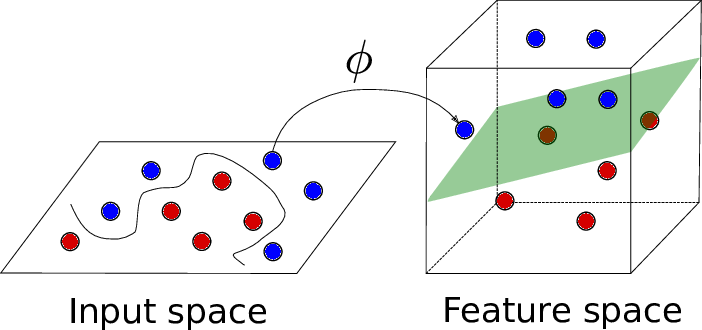Interpret as data scientist of the Probability density functions

Source: Deep Learning on MediumInterpret as data scientist of the Probability density functions

Random variable:

Descrete random variable: X is a discrete random variable, if its range is countable.

Continuous random variable: A continuous random variable is a random variable where the data can take infinitely many values. For example, a random variable measuring the time taken for something to be done is continuous since there is an infinite number of possible times that can be taken.

Population and sample:

• A population includes all of the elements from a set of data. mean of the population is denoted as μ.
• A sample consists of one or more observations drawn from the population. The mean of the sample is denoted as . if sampling was done randomly than it is called a random sample.

As sample size increases, the sample means converges to the population mean.

Depending on the sampling method, a sample can have fewer observations than the population, the same number of observations, or more observations. More than one sample can be derived from the same population.

Gaussian distribution(Normal distribution):

1. The mean, median and mode of the distribution coincide.
2. The curve of the distribution is bell-shaped and symmetrical about the line x=μ.
3. The total area under the curve is 1.
4. Exactly half of the values are to the left of the center and the other half to the right.

Most of the continuous random variables followed Gaussian distribution by nature. The probability density function can be shown below.

peak mostly located at the mean position of the population where σ² denoted variance of the population. σ² decides the shape of the PDF.

1. as x increases(move away from μ), y reduces exponential of the squire.
2. the curve is symmetric.
3. shape fall exponentially quadratic.

when mean = 0. all curve at probability =0.5.

as the variance decrease, the curve tries to become vertical line at x=0.

68–95–99.7 rule

68% of the points lie between -1σ to 1σ deviation of the mean.

Symmetric distribution, Skewness, and Kurtosis:

A symmetric distribution is a type of distribution where the left side of the distribution mirrors the right side. By definition, a symmetric distribution is never a skewed distribution.

1. Kurtosis measure the peakedness of a distribution.
2. Mean get impacted by outlier

The curve above the normal plot is positive kurtosis and below to the normal curve (N=0) is negative kurtosis.

Standard normal variate:

given any distribution with given points (X1,X2,X3,X4..) with mean and variance = N(μ,σ²), you can standardize to convert into standard normal variate N(0,1).

After standardization, you can tell simply the 68% of points lie between -1 and +1. and 95% point lies between -2 to +2.

Kernel density estimation:

Used to convert histogram into PDF.

take all heights of points on individual kernels and sum them. the sum is total height of distribution.

Sampling distribution & Central Limit theorem:

CLT: The means of each sample from the population is equal to the population mean(μ). the distribution comes from any distribution.

Quantile-Quantile plot(Q-Q plot):

To determine the random sample variables normally distributed or not. if the number of samples is small, it is had to interpret the Q-Q plot.

How distributions are used?

Gaussian distribution give the theoretical model of distribution of data which observed in many cases of natural phenomenon.

Suppose we know that data is distributed normally X ~ N (µ, σ) with mean µ and deviation σ. We can draw PDF and CDF using the above random data.

PDF and CDF tell us how data is distributed. PDF and CDF draw only in the case of Gaussian distribution.

Chebyshev’s inequality:

if I don’t know the distribution, mean=finite, and standard=finite. we can not draw PDF and CDF because of distribution.

here you can find %age of points lie between the given range.

Unform distribution:

it uses to generate a random number which has a lot of application. Height tells us what is probability of finding that value. The probability density function(PDF) for continuous random variable and probability mass function(PMF) for a discrete random variable.

NOTE: sample uniformly means each point have equal chance of lie in sample dataset D’

Log-Normal Distribution:

if ln(X) is normally distributed. if not, you can check using the Q-Q plot.

NOTE: if data given in log-normal, convert into Gaussian distribution by taking log. so you can use all ML techniques.

Most of the time in the real application, distribution is log-normal. Log-normal is right-skewed as we increase σ value. please see the example given below link.

example found at the below link.

Power law distribution:

also know as 80–20 rule. 80% of the time value found in a 20% interval.

Pareto distribution:

you can find an example in the application section in the above link.

Box cox transform:

if the dataset is in power-law/Pareto distribution, to convert into Gaussian distribution, use Box cox transform.

By putting all x value in Box cox function, you will get lambda( λ) value. use lambda( λ) value you can convert each x into y.

you can directly find Y value using the formula given in link

In a single line using boxcox(x) function, in just one line, we can find y value which is normally distributed.

Weibull distribution:

Used to measure the height of the dam. collect a one-week interval of rain data.

to determine particle size